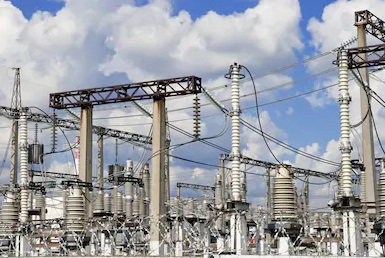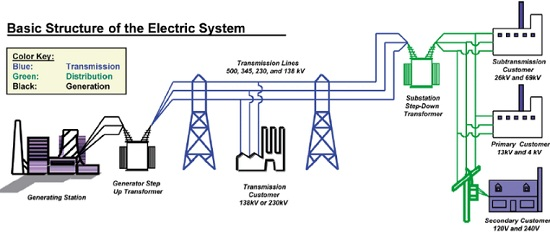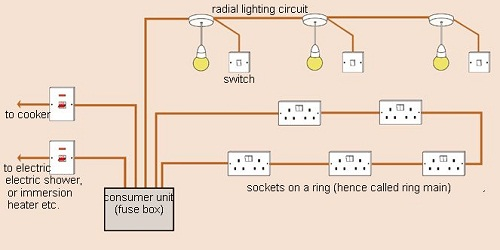# Main Electricity

### Guest Account

Attempt Form Four Physics Questions
Guest Account

# Main Electricity

- Mains electricity comes from a power station and its current is the alternating current which can either be stepped up or down by a transformer. A.c is produced when a conductor is rotated in a magnetic field or when a magnetic field is rotated near a conductor.
- This method is known as electromagnetic induction. The source of energy for rotating the turbine is the actual source of electrical energy. Most of the electricity in East Africa is generated from water.## Power transmission

This is the bulk transfer of electric power from one place to another. A power transmission system in a country is referred to as the national grid. This transmission grid is a network of power generating stations, transmission circuits and sub-stations. It is usually transmitted in three phase alternating current.

### Grid input

At the generating plant the power is produced at a relatively low voltage of up to 25 kV then stepped up by the power station transformer up to 400 kV for transmission. It is transmitted by overhead cables at high voltage to minimize energy losses. The cables are made of aluminium because it is less dense than copper. Metallic poles (pylons) carry four cables, one for each phase and the fourth is the neutral cable which is thinner and completes the circuit to the generator.

### Grid exit

At sub-stations transformers are used to step down voltage to a lower voltage for distribution to industrial and domestic users. The combination of sub-transmission (33 kV to 132 kV) and distribution (11 kV to 33 kV) which is then finally transformed to a voltage of 240 V for domestic use.### Electricity Distribution

This is the penultimate process of delivery of electric power. It is considered to include medium voltage (less than 50 kV) power lines, low voltage (less than 1,000 V) distribution, wiring and sometimes electricity meters.

#### Dangers of high voltage transmission

1. They can lead to death through electrocution
2. They can cause fires during upsurge
3. Electromagnetic radiations from power lines elevate the risk of certain types of cancer

### Electrical power and energy

Work done = volts × coulombs = VQ, but Q = current × time = I t.
So work done = V I t
Other expressions for work may be obtained by substituting V and I from Ohms law as below
V = I R and I = V / R, work done = I R × I t = I2 R t, or work done = V × V t / R = V2t / R.

The three expressions can be used to calculate work done. Electrical power may be computed from the definition of power.
Power = work / time = I2R t /t = I2 R or V2t / R t = V2/ R
Using work done = V I t, then Power = V I.

These expressions are useful in solving problems in electricity. Work done or electrical energy is measured in joules (J) and power is measured in watts (W). 1 W = 1 J/s.

#### Example

An electric heater running on 240 V mains has a current of 2.5 A.
1. What is its power rating?
2. What is the resistance of its element?
Solution
1. Power = V I = 240 × 2.5 = 600 W. Rating is 600 W, 240 V.
2. Power = V / R = 600 W. R = V / I. R = 240 / 2.5 = 96 Ω.

### Costing Electricity

The power company uses a unit called kilowatt hour (kWh) which is the energy transformed by a kW appliance in one hour. 1 kW = 1,000 W × 60 × 60 seconds = 3,600,000 J. The meter used for measuring electrical energy uses the kWh as the unit and is known as joule meter.

#### Examples

1. An electric kettle is rated at 2,500 W and uses a voltage of 240 V.
a) If electricity costs Ksh 1.10 per kWh, what is the cost of running it for 6 hrs?
b) What would be its rate of dissipating energy if the mains voltage was dropped to 120 V?
Solution
a) Energy transformed in 6 hrs = 2.5 × 6 = 15 kWh. Cost = 15 × 1.10 × 6 = Ksh 99.00
b) Power = V2/ R = 2500. R = (240 × 240) /2500 = 23.04 Ω.
Current = V / R = (240 × 2500) / (240 × 240) = 10.42 A
Power = V I = (2500 × 120) / 240 = 1,250 W.

2. An electric heater is made of a wire of resistance 100 Ω connected to a 240 V mains supply. Determine the;
a) Power rating of the heater
b) Current flowing in the circuit
c) Time taken for the heater to raise the temperature of 200 g of water from 23°C to95°C. (specific heat capacity of water = 4,200 J Kg-1K-1)
d) Cost of using the heater for two hours a day for 30 days if the power company charges Ksh 5.00 per kWh.
Solution
a) Power = V2/ R = (240 × 240) / 100 = 576 W
b) P = V I =>> I = P / V = 576 / 240 = 2.4 A
c) P × t = heat supplied = (m c θ) = 576 × t = 0.2 × 4200 × 72.
Hence t = (0.2 × 4200 × 72) / 576 = 105 seconds.
d) Cost = kWh × cost per unit = (0.576 × 2 × 30) × 5.0 = Ksh 172.80

3. A house has five rooms each with a 60 W, 240 V bulb. If the bulbs are switched on fro 7.00 pm to 10.30 pm, calculate the;
a) Power consumed per day in kWh
b) Cost per week for lighting those rooms if it costs 90 cents per unit.
Solution
a) Power consumed by 5 bulbs = 60 × 5 = 300 W = 0.3 kWh. Time = 10.30 – 7.00 = 3½
hrs.Therefore for the time duration = 0.3 × 3½ = 1.05 kWh
b) Power consumed in 7 days = 1.05 × 7 = 7.35 kWh. Cost = 7.35 × 0.9 = Ksh 6.62

### Domestic wiring system

- Power is supplied by two cables where one line is live wire (L) and the other is neutral (N).
- Domestic supply in Kenya is usually of voltage 240 V. The current alternates 50 times per second hence the frequency is 50 Hz. The neutral is earthed to maintain a zero potential. - The main fuse is fitted on the live wire to cut off supply in case of a default. A fuse is a short piece of wire which melts if current of more value flows through it.
- Supply to the house is fed to the joule meter which measures the energy consumed.
- From the meter both L and N cables go to the consumer box (fuse box) through the main switch which is fitted on the live cable.
- Consumer units within the house are fitted with circuit breakers which go off whenever there is a fault in the system.
- Lights in the house are controlled by a single or double switch (two way).
- In most wiring systems the main sockets are connected to a ring main which is a cable which starts and end at the consumer unit. Plugs used are the three-pin type.